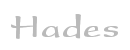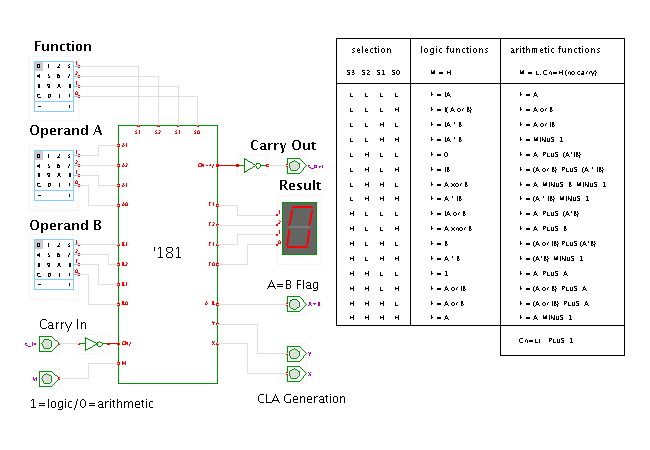TAMS / Java / Hades / applets (print version): contents | previous | next

#### TTL-series 74181 ALU demonstrationDescription

This applet demonstrates the TTL-series 4-bit 74181 arithmetic-logical unit. It takes 4-bit operands and a carry input and calculates one of 16 logical or 16 arithmetic functions. Two additional active-low outputs X (/propagate) and Y (/generate) allow to interface to the 74182 carry-lookahead generator IC for fast addition.

The M input selects whether the ALU should calculate logical functions (M=1) or arithmetic functions (M=0). In each mode, the four S3..S0 opcode inputs select one of the available operations; check the table in the applet schematics for the specific funtions selected by each opcode. For example, M=0 and S=(1001) select the normal binary addition including carry, Z=(A+B+Cin).

Run the applet | Run the editor (via Webstart)

Impressum | 24.11.06